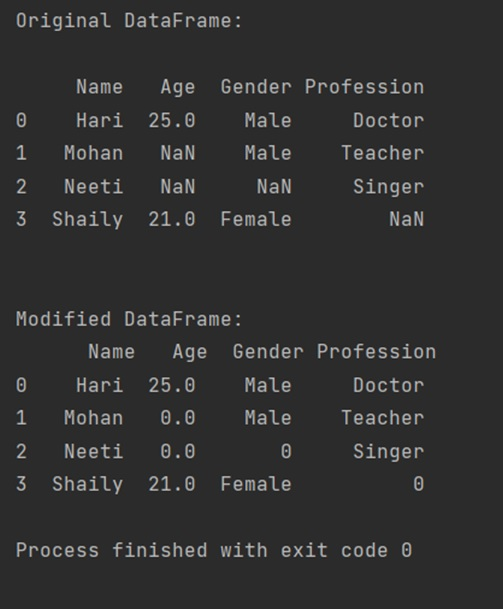# How to Replace NaN Values with Zeros in Pandas DataFrame?

Replace NaN with Zeros: In this tutorial, we will learn how to replace NaN values with zeros in Pandas DataFrame? By Pranit Sharma Last updated : April 19, 2023

## Overview

While creating a DataFrame or importing a CSV file, there could be some NaN values in the cells. NaN values mean "Not a Number" which generally means that there are some missing values in the cell. To deal with this type of data, you can either remove the particular row (if the number of missing values is low) or you can handle these values.

## Replace NaN with Zeros in Pandas DataFrame

To replace NaN values with zeroes in a Pandas DataFrame, you can simply use the DataFrame.replace() method by passing two parameters to_replace as np.NaN and value as 0. It will replace all the NaN values with Zeros.

Let's understand with the help of Python program.

## Python Program to Replace NaN Values with Zeros in Pandas DataFrame

In the below example, there is a DataFrame with some of the values and NaN values, we are replacing all the NaN values with zeros (0), and printing the result.

```# Importing pandas package
import pandas as pd

# To create NaN values, you must import numpy package,
# then you will use numpy.NaN to create NaN values
import numpy as np

# Creating a dictionary with some NaN values
d = {
"Name":['Hari','Mohan','Neeti','Shaily'],
"Age":[25,np.NaN,np.NaN,21],
"Gender":['Male','Male',np.NaN,'Female'],
"Profession":['Doctor','Teacher','Singer',np.NaN]
}

# Now, Create DataFrame
df = pd.DataFrame(d)

# Printing the original DataFrame
print("Original DataFrame:\n")
print(df,"\n\n")

# Replacing NaN values with 0
df = df.replace(np.nan, 0)

# Printing The replaced vales
print("Modified DataFrame:\n",df)
```

### Output